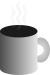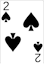#Coffee SpaceListen:

## Other Dimensions

TL;DR: This is some random thinking in my mind currently I thought that I would write down. It has no basis in reality as such, just some brain farts. It should not be taken seriously. It could just be a good point to start a conversation.

### Fourth Dimension

A form of the fourth dimension appears to exist due to our observations with quantum mechanics, but it doesn’t appear to exist in the way we think of three dimensions.

In games or physics, we can describe a location as $\{ x, y, z \}$ and another location relative to this point describes rotation $\{ a, b, c \}$. The distance between the two points, i.e. the magnitude described by:

$d = \sqrt{ (x - a)^2 + (y - b)^2 + (z - c)^2}$

It’s unclear to me what this distance would represent in the physical world, but it could represent something, such as time.

If we had an additional fourth dimension, you might think it would be $\{ w, x, y, z \}$ for location and $\{ a, b, c, d \}$ to encode another point to give rotation, so for distance we have:

$d = \sqrt{ (w - a)^2 + (x - b)^2 + (y - c)^2 + (z - d)^2}$

The problem with this is, if the fourth dimension also had a spatial and rotation element, you would think that nature would make use of this? Physical things would be happening in this space and it could be leveraged in some way. And yet, we don’t. We have no sensors to perceive it, no actions to act in it, and how brains are not well developed to even think about it. It seems to indicate that it doesn’t exist in both a spatial and rotation setting.

It appears that the fourth dimension only has rotation and not spatial properties, but it’s not obvious how these present themselves, and I don’t really have the math skills to back up my thinking.

It may look something like $\{ f(x, y, z), x, y, z \}$ for location (note the location of the 4th dimension is derived by some unknown function $f()$ based on three dimensional spatial data $x, y, z$) and $\{ a, b, c, d \}$ for rotation encoding. For distance we would have:

$d = \sqrt{ (f(x, y, z) - a)^2 + (x - b)^2 + (y - c)^2 + (z - d)^2}$

#### 4+ Dimensions

It is not clear how they may stack if more exist after this. Five dimensions could be represented as:

$d = \sqrt{ g(f(x, y, z), x, y, z), (f(x, y, z) - a)^2 + (x - b)^2 + (y - c)^2 + (z - d)^2}$

Meaning that the 5th dimension function $g()$ is some function of the lower dimensions. It could also be:

$d = \sqrt{ g(x, y, z), (f(x, y, z) - a)^2 + (x - b)^2 + (y - c)^2 + (z - d)^2}$

Where $g()$ is only based on three dimensions or $g()$ could equal $f()$.

#### Space Filling Curve

By far the more interesting idea to me is that there is one fundamental dimension, with all other dimensions being some function of this. In this case I will denote $S$ as a spatial representation, such that $S = \{ s_0, .., s_n \}$ and the rotation properties as $R = \{ r_0, .., r_n \}$. Each spatial function is also now $F = \{ f_0(), .., f_n() \}$ and each rotation function is now $G = \{ g_0(), .., g_n() \}$. This would give us:

$d = \sqrt{ (f_0(s_0), g_0(r_0))^2 + (f_1(f_0(..), s_1), g_1(g_0(..), r_1))^2 + .. +}$

$\overline{(f_n(f_{n-1}(..), s_n), g_n(g_{n-1}(..), r_n))^2 }$

It could be that there is a 4th or higher spatial dimension, but it is so diluted that it becomes undetectable compared to the first three dimensions. It is unclear what $f()$ and $g()$ may look like.

As for showing that at least two rotational properties $R$ are calculated based on one-another, see the gimbal lock problem. They are clearly not independent variables.

In terms of spatial dimensions being intertwined, it’s entirely possible there is some redundancy here too, if there is limits to each spatial axis (I think the idea of the Universe being infinitely large is insane if at some point it was condensed into single point and has not inflated infinitely fast). It’s entirely possible that the Universe is spatially some form of torus or similar type of shape where the edges wrap around on themselves.

Wrapping two dimensional space would be a sphere as shown here:

There appear to be several available options in four dimensional space, although these become increasingly difficult to both model and visualize. Something worth noting though is when we go from a 3D torus surface equation:

$r^2 = (\sqrt{x^2 + y^2} - R)^2 + z^2$

To a 4D Ditorus surface equation:

$R^2 = (\sqrt{(\sqrt{x^2 + y^2} - p)^2 + z^2} -r)^2 + w^2$

Where $R$ is the major-major radius, $r$ is the major-minor radius and $p$ is the minor-minor radius. Read more here. These can be visualized as follows:

Something to note is that some of those dimensions are more influential than others, as we increase the number of dimensions beyond four, some of the smaller spatial dimensional terms have diminishing impact to a given 3D coordinate space $(x, y, z)$. Evolution wise, it makes no sense to even begin to try and model near unobservable properties you neither have the sensors or actuators to deal with.
Time is the measurements of events per tick, where ticks is some universal constant time frame (this would be constant no matter location of place in the life of the Universe) and events are some real positive value. Time is simply an observation of the number of events that occurred, a negative number of events cannot ever occur, hence time is always a value $\ge 0$.